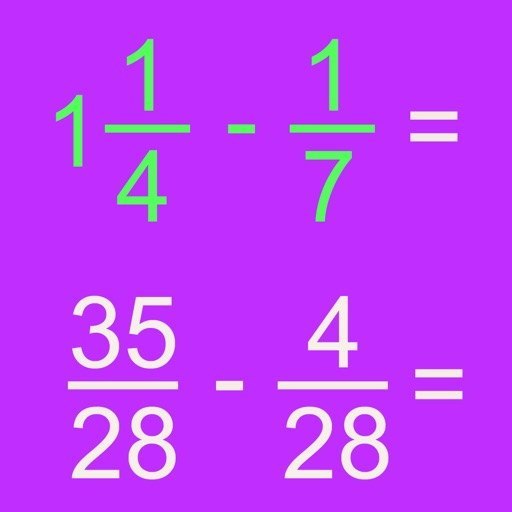## The Fraction Math app can be used to teach and study fractions by solving addition, subtraction, division and multiplication problems with fractions step by step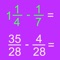# Fraction Math

by Esa Helttula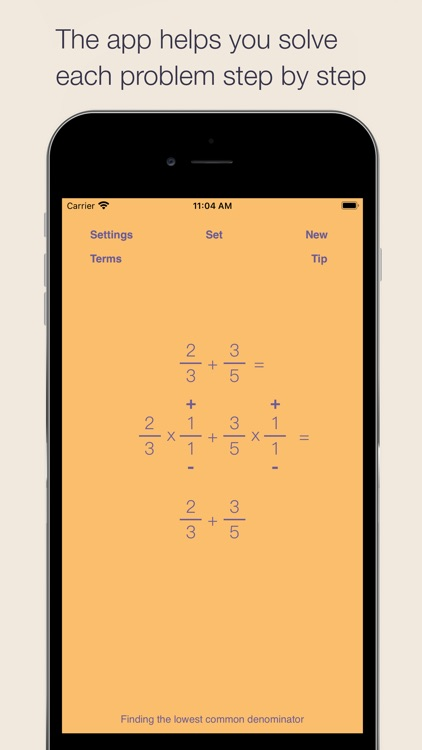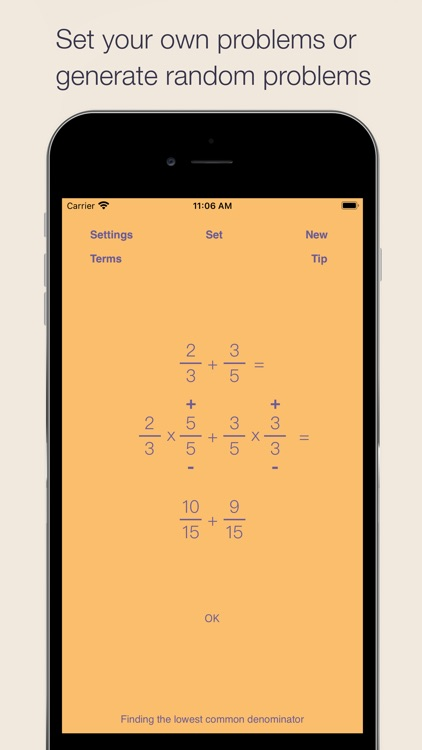\$3.99 in the App Store

Fraction Math is exactly what the title says. It's an app about fractions. The lessons are detailed and it is full of content, but the UI is very similar to a standardized test, which keeps it from being an essential app. Because of its resemblance to a test, it may not be the best app to engage your young mathematician.The Fraction Math app can be used to teach and study fractions by solving addition, subtraction, division and multiplication problems with fractions step by step. Wired.com: "Quite a useful app for learning many fraction computations."

Version
6.5
Rating
(4)
Size
8Mb
Genre
Education
Last updated
October 4, 2021
Release date
May 19, 2010

### App Screenshots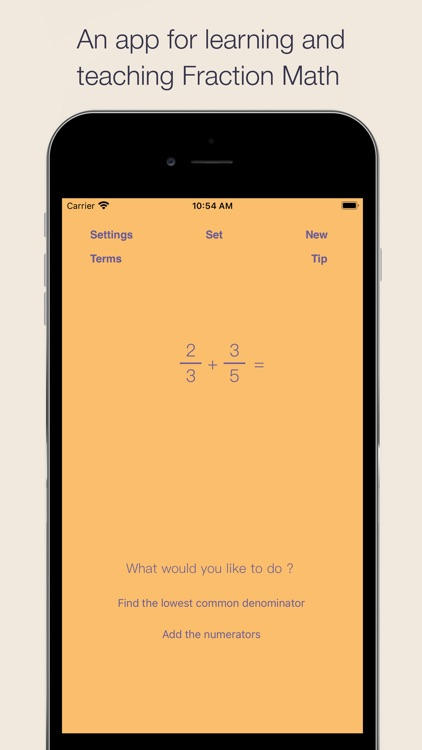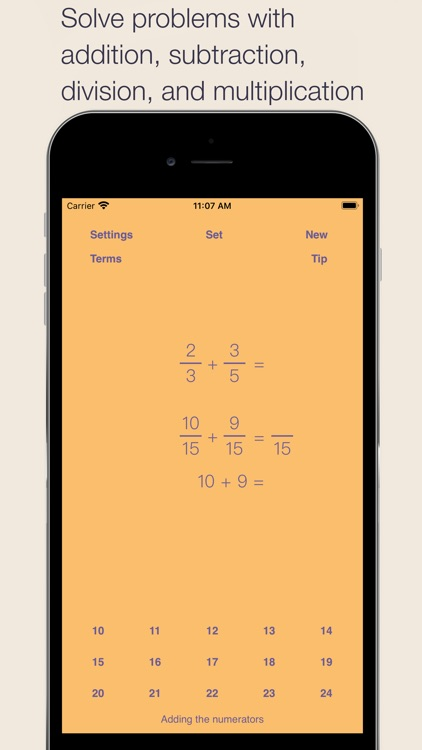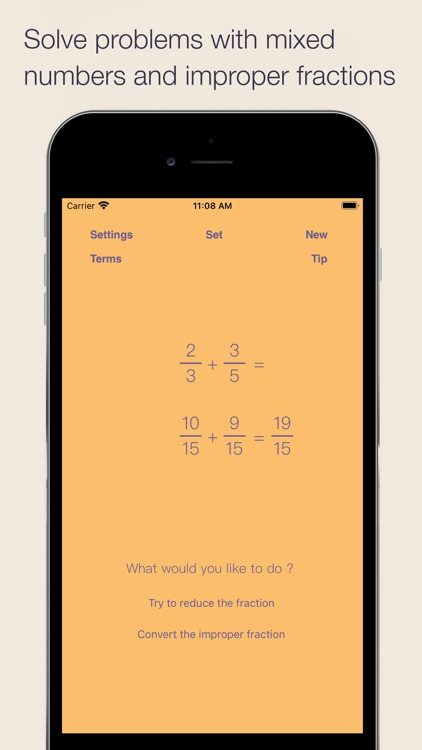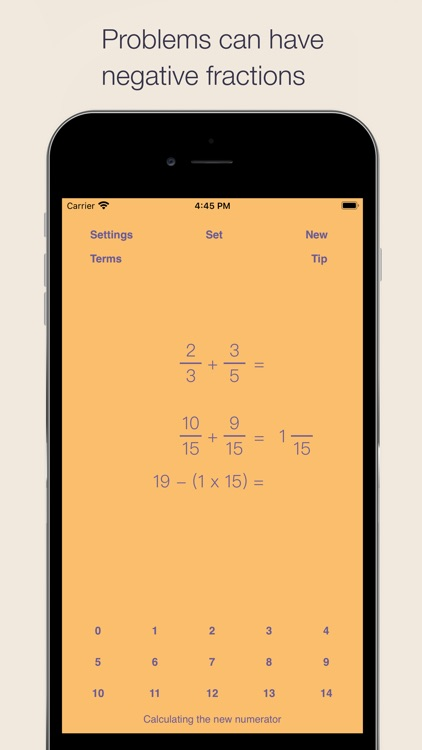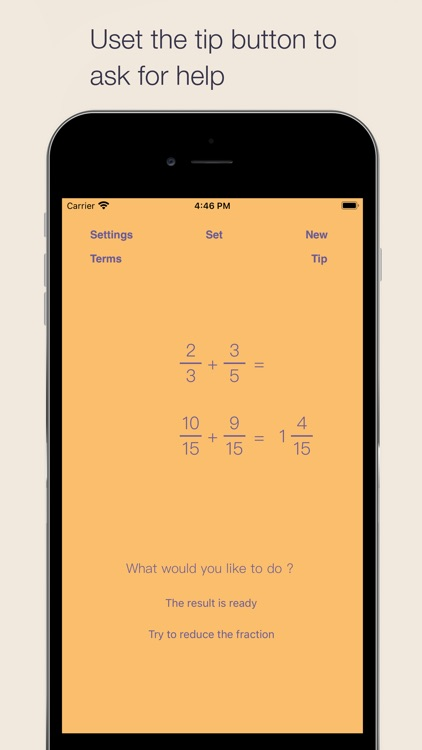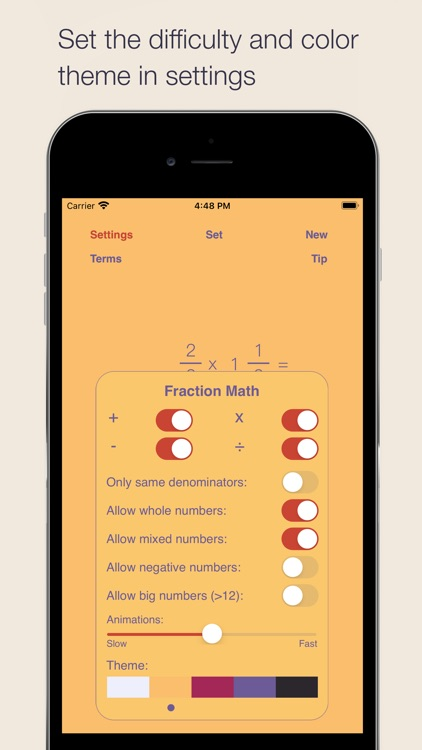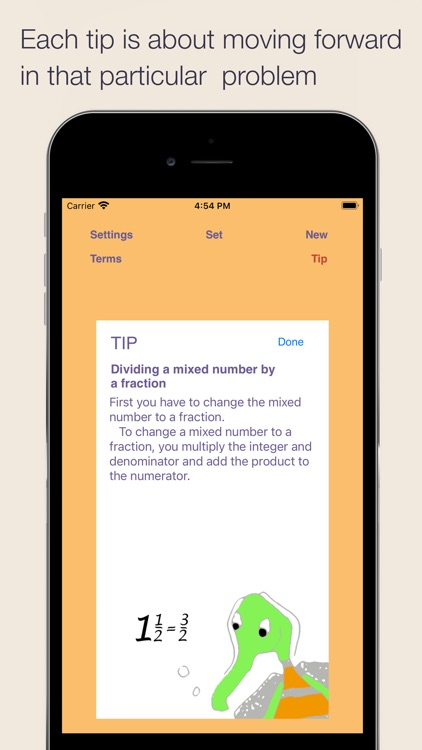### App Store Description

The Fraction Math app can be used to teach and study fractions by solving addition, subtraction, division and multiplication problems with fractions step by step. Wired.com: "Quite a useful app for learning many fraction computations."

The Fraction Math app can handle positive and negative fractions, improper fractions, whole numbers and mixed numbers. The user can also reduce the resulting fractions.

The user can input his/her own fractions, mixed numbers, and whole numbers. The app has also an illustrated glossary of the most common terms.

Recommended by reviewers

Wired.com:

"Quite a useful app for learning many fraction computations."

Fun Educational Apps:

"Fraction Math app is easy to use and really takes you step by step when adding, subtracting or multiplying fractions."

A third-grade student can use the app to add and subtract fractions with equal denominators.

A fourth-grade student can use the app to add or subtract fractions and mixed numbers with different denominators and also to reduce the resulting fractions.

A fifth-grade student can multiply fractions by whole numbers and other fractions. She can also divide fractions and mixed numbers.

A sixth or higher-grade student can use negative fractions, whole numbers and mixed numbers in the problems. She can also use larger numbers.

The above grades and their uses of the app are only provided as examples.

The user can choose how to solve the problems

In each step the user is presented with two choices. Sometimes both of the choices are right. When multiplying fractions the user can choose to first multiply the numerators or the denominators.

In those cases when there is only one possible next step, the user can choose between the right step and a random wrong step. For wrong steps, the app will tell, why that is the wrong step.

Intelligent help system

The Fraction Math app has a help system that provides over 60 context sensitive tips based on the state of the app. For example, when searching for a lowest common denominator, the help system will first tell how to search for the answer. If, after trying herself the user cannot find the answer, the help system will tell her what the lowest common denominator is.

Smart keyboard

When the user has to enter numbers, the Fraction Math app uses the same intuitive smart rolling keyboard as my other apps. Only one tap is needed to enter any number. In reducing fractions, a special prime number keyboard is shown.

Every step is animated

Every step in solving the problems is animated. The speed of the animations can be set and they can also be turned off.

Fun illustrations

The 60 different tips have a lot of information about fractions, mixed numbers, improper fractions and reduction of fractions. The tips have over 50 illustrations to keep the user interested in reading the tips.

The app has the following settings:

- allow subtraction
- allow multiplication
- allow division
- only allow fractions with same denominators
- allow whole numbers
- allow mixed numbers
- allow negative numbers
- allow numbers grater than 12

Other iDevBooks math apps

iDevBooks math apps have been reviewed and endorsed by Wired.com, IEAR.org, Edudemic.com, Teachers with Apps, and other respected sites and organizations.

Some of the other 40 iDevBooks math apps are Column Addition, Long Multiplication, Long Division, Column Subtraction, Lattice Multiplication, Visual Multiplication Table, Partial Differences Subtraction, Partial Quotients Division, Partial Sums Addition, Decimal Rounding, etc.

Feedback and requests for new features

New ideas to make this app better are welcome. Please visit idevbooks.com to give feedback.

Privacy

This app has no ads or in-app purchases and it does not transmit any data during the operation of the app. This app also does not contain any links to other apps or the web.

Disclaimer:
AppAdvice does not own this application and only provides images and links contained in the iTunes Search API, to help our users find the best apps to download. If you are the developer of this app and would like your information removed, please send a request to takedown@appadvice.com and your information will be removed.